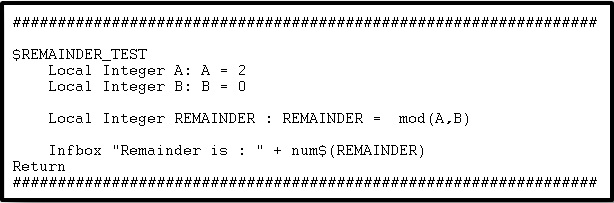# How to calculate remainder using x3 mod function

By | October 30, 2014

You may come across the situation, where you having arithmetic operation with numbers. You may want to calculate the remainder of division.

Sage X3 has a special function to calculate the remainder of division.

mod: This function calculates the remainder of division.

Syntax:  mod(num_exp1, num_exp2)

Parameter details:

num_exp1 and num_exp2 are two numbers used in division.

Kindly refer to the below snippet of code for better understanding